# Accessing elements of a Pandas Series

• Difficulty Level : Basic
• Last Updated : 17 Jan, 2019

Pandas Series is a one-dimensional labeled array capable of holding data of any type (integer, string, float, python objects, etc.). Labels need not be unique but must be a hashable type. An element in the series can be accessed similarly to that in an ndarray. Elements of a series can be accessed in two ways –

• Accessing Element from Series with Position
• Accessing Element Using Label (index)In this article, we are using “`nba.csv`” file, to download the CSV, click here.

### Accessing Element from Series with Position

In order to access the series element refers to the index number. Use the index operator [ ] to access an element in a series. The index must be an integer.
In order to access multiple elements from a series, we use Slice operation. Slice operation is performed on Series with the use of the colon(:). To print elements from beginning to a range use `[:Index]`, to print elements from end-use` [:-Index]`, to print elements from specific Index till the end use `[Index:]`, to print elements within a range, use [Start Index:End Index] and to print whole Series with the use of slicing operation, use `[:]`. Further, to print the whole Series in reverse order, use` [::-1]`.

Code #1: Accessing a first element of series

 `# import pandas and numpy ``import` `pandas as pd``import` `numpy as np`` ` `# creating simple array``data ``=` `np.array([``'g'``, ``'e'``, ``'e'``, ``'k'``, ``'s'``, ``'f'``, ``'o'``, ``'r'``, ``'g'``, ``'e'``, ``'e'``, ``'k'``, ``'s'``])``ser ``=` `pd.Series(data)``  ` `  ` `# retrieve the first element``print``(ser[``0``])`

Output :

```g
```

Code #2: Accessing first 5 elements of Series

 `# import pandas and numpy ``import` `pandas as pd``import` `numpy as np`` ` `# creating simple array``data ``=` `np.array([``'g'``, ``'e'``, ``'e'``, ``'k'``, ``'s'``, ``'f'``, ``'o'``, ``'r'``, ``'g'``, ``'e'``, ``'e'``, ``'k'``, ``'s'``])``ser ``=` `pd.Series(data)``  ` `  ` `# retrieve the first element``print``(ser[:``5``])`

Output :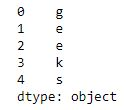Code #3: Accessing last 10 elements of Series

 `# import pandas and numpy ``import` `pandas as pd``import` `numpy as np`` ` `# creating simple array``data ``=` `np.array([``'g'``, ``'e'``, ``'e'``, ``'k'``, ``'s'``, ``'f'``, ``'o'``, ``'r'``, ``'g'``, ``'e'``, ``'e'``, ``'k'``, ``'s'``])``ser ``=` `pd.Series(data)``  ` `  ` `# retrieve the first element``print``(ser[``-``10``:])`

Output :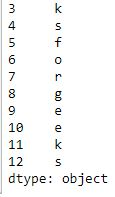Code #4: Accessing first 5 elements of Series in `nba.csv` file

 `# importing pandas module  ``import` `pandas as pd  ``     ` `# making data frame  ``df ``=` `pd.read_csv(``"nba.csv"``)  ``   ` `ser ``=` `pd.Series(df[``'Name'``]) ``ser.head(``10``) `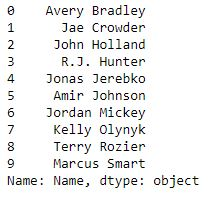Now we access first 5 elements of series

 `# get first five names ``ser[:``5``] `

Output :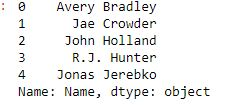### Accessing Element Using Label (index)

In order to access an element from series, we have to set values by index label. A Series is like a fixed-size dictionary in that you can get and set values by index label.

Code #1: Accessing a single element using index label

 `# import pandas and numpy ``import` `pandas as pd``import` `numpy as np`` ` `# creating simple array``data ``=` `np.array([``'g'``, ``'e'``, ``'e'``, ``'k'``, ``'s'``, ``'f'``, ``'o'``, ``'r'``, ``'g'``, ``'e'``, ``'e'``, ``'k'``, ``'s'``])``ser ``=` `pd.Series(data, index ``=``[``10``, ``11``, ``12``, ``13``, ``14``, ``15``, ``16``, ``17``, ``18``, ``19``, ``20``, ``21``, ``22``])``  ` `  ` `# accessing a element using index element``print``(ser[``16``])`

Output :

```o
```

Code #2: Accessing a multiple element using index label

 `# import pandas and numpy ``import` `pandas as pd``import` `numpy as np`` ` `# creating simple array``data ``=` `np.array([``'g'``, ``'e'``, ``'e'``, ``'k'``, ``'s'``, ``'f'``, ``'o'``, ``'r'``, ``'g'``, ``'e'``, ``'e'``, ``'k'``, ``'s'``])``ser ``=` `pd.Series(data, index ``=``[``10``, ``11``, ``12``, ``13``, ``14``, ``15``, ``16``, ``17``, ``18``, ``19``, ``20``, ``21``, ``22``])``  ` `  ` `# accessing a multiple element using ``# index element``print``(ser[[``10``, ``11``, ``12``, ``13``, ``14``]])`

Output :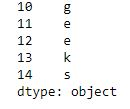Code #3: Access multiple elements by providing label of index

 `# importing pandas and numpy  ``import` `pandas as pd  ``import` `numpy as np ``   ` `ser ``=` `pd.Series(np.arange(``3``, ``9``), index ``=``[``'a'``, ``'b'``, ``'c'``, ``'d'``, ``'e'``, ``'f'``]) ``   ` `print``(ser[[``'a'``, ``'d'``, ``'g'``, ``'l'``]])`

Output :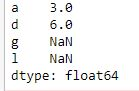Code #4: Accessing a multiple element using index label in `nba.csv` file

 `# importing pandas module  ``import` `pandas as pd  ``     ` `# making data frame  ``df ``=` `pd.read_csv(``"nba.csv"``)  ``   ` `ser ``=` `pd.Series(df[``'Name'``]) ``ser.head(``10``) `Now we access an multiple element using index label

 `ser[[``0``, ``3``, ``6``, ``9``]] `

Output :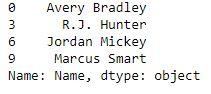My Personal Notes arrow_drop_up